#### Discounted Cash Flow

Discounted cash flow is the foundation on which all other valuation approaches are built. This method is commonly used by practitioners to derive a conclusion of value of an asset as part of the income approach, which is one of the three ways of approaching a business valuation. In this short article, we will explain the basics of this method and provide examples on how it can be built.

## What is Discounted Cash Flow (DCF)?

The discounted cash flow method is one of the methods used to derive the value of an asset within the income approach. This method’s foundation is in the present value rule, where the value of any asset is the present value of the expected future cash flows it will produce (based on the cash flows the asset will produce in the future, and what the equivalent value of those future cash flows expressed in dollars is as of today). In order to derive the present value, the method uses an appropriate discount rate that represents the risk an investor is willing to accept for the potential reward an investment in the subject asset will return. Different assets derive different risk profiles, and the lower the expected risk, the lower the expected return, from government bonds (0-2%) to the stock market (5-8%) to the majority of private businesses (10-30%).

An easy way to understand the DCF analysis is through the two-stage model, which can be summarized in the following seven steps:

Step 1: Determine a number of periods for which projections of net cash flowscan be made (commonly, the number of periods used are five to ten years).

• Step 2: Estimate the net cash flowthat would be generated in each period in the projections. This gives flexibility to forecast specific events, like buying a second store, launching a new product, or the opposite—stopping a revenue line because of bad performance.
• Step 3: Estimate a perpetual growth rate in net cash flowsfrom the end of the projected period moving forward. Once a perpetual growth rate is selected, generally at the five- or ten-year mark into the future, very little certainty/confidence can be given to specific forecasts, so one perpetual growth rate is selected, generally aligning close to the wider economy GDP growth or inflation numbers.
• Step 4: Use the constant growth model (there are different models, but the most commonly used is the Gordon Growth model) to estimate the present value of the net cash flowsexpected into perpetuity as of the end of the projection period based on the perpetual growth rate.
• Step 5: Select a discount rate (quantifying the risk/return) to “discount” the cash flowsto the present value. This is a complex step that requires a valuation professional to get it right. To give you a guide, public companies on the stock market can often use discount rates of 5-8%, whilst most small private businesses fall between 10-30%.
• Step 6: Discount each of the net cash flowsback to their present value using the discount rate including:
• Step 7: Sum the value derived from Step 6.

Sometimes, the projected periods’ growth rate in revenue or net cash flow differ greatly to the perpetual growth rate used to determine the terminal value. Consequently, some argue that this is unlikely to happen in the practice. Instead, it’s considered closer to reality for the growth to gradually decrease into a perpetual growth rate.

The next formula summarizes the basic DCF framework.

Basic DCF Framework (Two-Stage Model)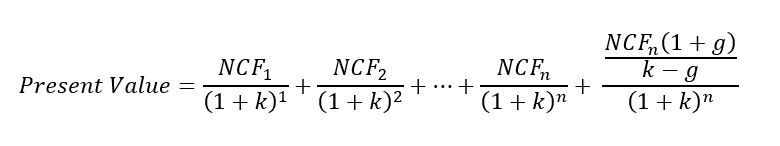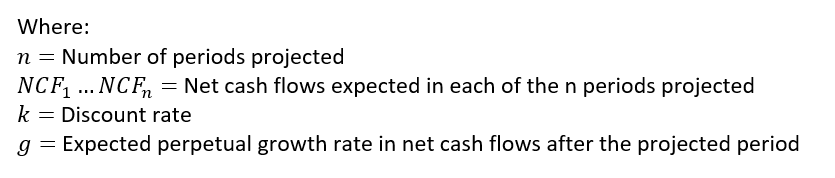DCF model

Below is an illustration on how a two-stage model could be built in the case of finding the total present value of the future expected net cash flows of a company.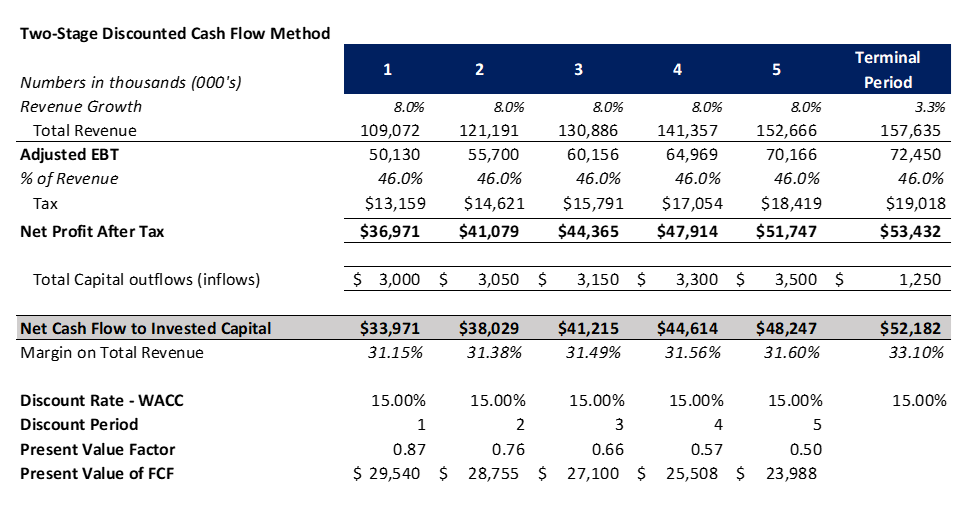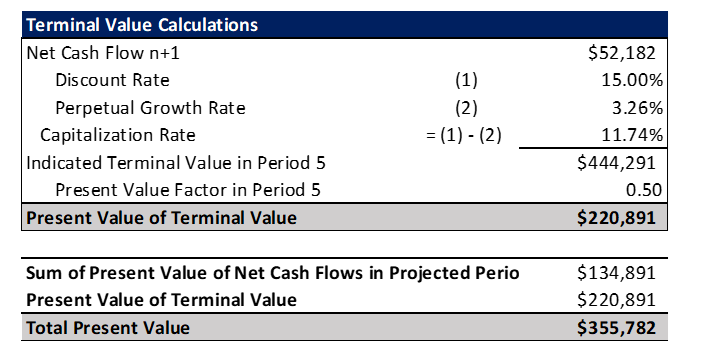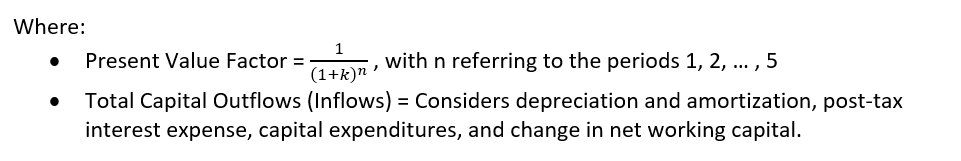The example finds the future expected net cash flows to invested capital (those available to both equity holders and debt investors). These net cash flows in the projected period are then brought to their present value with a discount rate and summed together. It then finds the net cash flow in the terminal period and uses a capitalization rate as calculated above to derive a terminal value, which is also brought to the present value using the discount rate. The final value of the company is the total present value of the expected future net cash flow that the firm is estimated to generate during the explicit forecast period and beyond the explicit forecast period.

In the example, you can see the sum of \$134,891 and \$220,891 for \$355,782.

Note: this example was made for illustration purposes only, and the assumptions and numbers used in the model are completely fictious.

## Benefits of DCF Analysis

The DCF analysis is one of the preferred choices by analysts to derive the value of a firm. However, it depends on different case facts and capabilities to estimate future expected net cash flows. Some of the benefits of the DCF analysis:

extremely detailed

• based on the foundations of valuation
• allows one to build assumptions regarding key value drivers of a business
• can be used to determine the values of different assets (e.g., companies, individual projects)
• allows flexibility to build different scenarios of assumptions
• suitable for most of the valuation purposes, such as tax planning, potential sales, strategic planning, etc.

## Cons of DCF analysis

While the DCF analysis has many advantages, any model used to estimate a value is prone to potential errors, which include:

sensitivity to assumptions

• it looks at the company value in isolation
• the constant growth model assumption can be unrealistic
• limited usability when the asset is not yet cash flow positive
• detailed assumptions into the future can be very hard to predict

## Conclusion

The discounted cash flow analysis is a powerful tool to determine the value of an asset that is able to generate positive cashflows. It’s commonly used in different areas associated to finances, and is one of the primary models taught in academia. Its application is tied to the foundations of valuing an asset and, despite some flaws, it’s a highly regarded technique by many professionals.Author